# PV in ExcelArticle byExcelMojo Team## What Is PV Excel Function?

The PV in Excel stands for the Present Value. In simple terms, the Present Value function calculates the present value of investments. It is calculated on the discount rate, number of periods of investments, and future value of the payment.

Generally, PV is used in financial projects. Also, it is used to find the current value of a future stream of payments. For example, there is an investment of \$10,000 in ABC Bank’s bond, and the interest rate is 10% for ten years. Now, let us learn how to find PV in Excel.

The steps used to calculate PV in Excel are as follows:

• First, enter the PV formula in cell B5 to calculate the Present Value of Investment. So, the entered formula is =PV(B2,B4,B3,0,0).
• Next, press Enter key.
• Clearly, we can see the result in cell B5.

Likewise, we can calculate the value using PV in excel.

###### Key Takeaways
• The PV in Excel calculates the Present Value of the investments made by the investor in the form of shares, bonds, debentures, etc.
• The formula of PV in excel is =PV(rate,nper,pmt,[fv],[type])
• Here, rate, nper, pmt are mandatory arguments. They denote the rate of interest, number of periods of annuity or investment, and the payment made per period with the principal amount and interest, respectively.
• The fv and type are optional arguments and indicate the Future Value of annuity at the end of payments and the payment made from the start or end of the period, respectively.

### PV( ) Excel Formula

The syntax of the PV Excel Function is

where,

• rate: It is a mandatory argument and denotes the rate of interest or discount rate.
• nper: It indicates the number of periods of annuity or investment and is a mandatory argument.
• pmt: It is also a mandatory argument, representing the payment made per period with principal amount and interest.
• fv: It is an optional argument that defines the Future Value of annuity at the end of payments.
• type: It is also an optional argument, showing that the payment is made from the start or end of the period.

The value is 0 or 1;

0 is used to show that the payment was made at the end, and 1 denotes payment made at the start of the period.

### How To Calculate Present Value In Excel? (With Steps)

We can calculate Present Value in Excel using the below two methods:

1. Access PV Function From The Excel Ribbon

1. First, go to the Formulas tab.

2. Next, click on the Financial option from the Function Library group.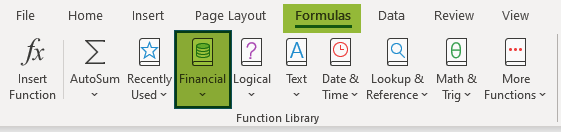3. Then, choose the PV function from the drop-down list.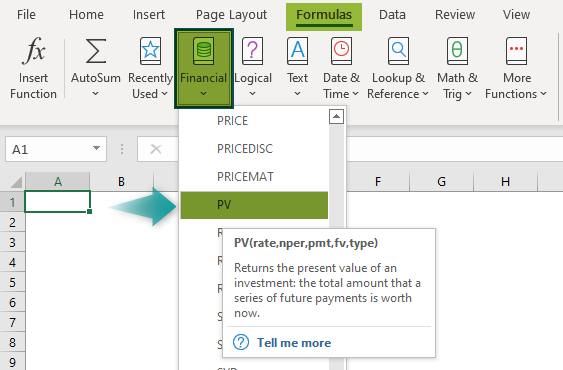4. The Function Arguments window pops up.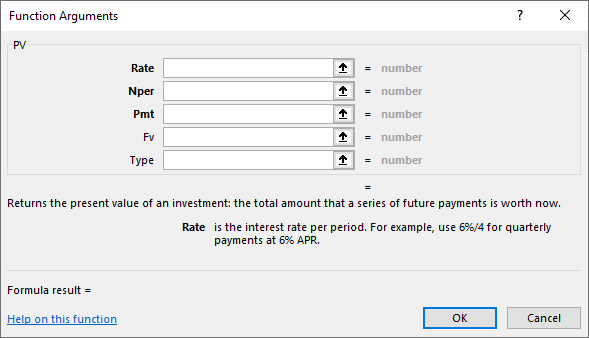5. Now, enter the arguments in the Rate, Nper, Pmt, Fv & Type box.

6. Click OK.

2. Enter The Worksheet Manually

• To begin with, select an empty cell for the output.
• Next, type =PV( in the selected cell. Alternatively, type =P and double-click the PV function from the list of suggestions shown by Excel.

Then, enter the arguments as cell references or direct values. Close the parenthesis and press the Enter key.

Let us take a basic example of how to use PV in Excel. Mr. Andy has invested \$5000 in a company’s bond; the interest rate for 5 years is 5%. Now, let us calculate PV in excel.

In the table below,

• Column A contains investments.
• Column B contains data.

The steps to calculate PV are as follows:

Step 1:  First, select an empty cell to display the output. We have selected cell B5 in this case.

Step 2: Next, enter the formula in cell B5. Then, select the cell that contains the rate, i.e., ‘B2’ for the Rate argument.

Step 3: Now, we need to select the cell that contains the number of periods for the second argument.

So, in our example, we should select cell B4.

Step 4: Next, we should select the cell that contains the investment per period in the third argument. In our example, we should select cell B3.

Step 5: Now, we should enter the future value in the fourth argument. So, type ‘0’.

Step 6: Then, enter the period type, i.e., ‘0-end of the period.’

So, the complete formula is =PV(B2,B4,B3,0,0).

Step 7: Next, press the Enter key.

Clearly, we can see the results in cell B5 as \$21,647.38, as shown in the image below.

Thus, we can calculate amount using PV in excel.

### Examples

Let us look at the following examples to understand the method to calculate PV in excel.

#### Example #1 – PV Of Annuity

Let us assume that the monthly installment is \$2,000, the interest rate for 5 years is 10%, and the payment per year is 12. Now, let us learn how to find PV in Excel with monthly instalments.

In the table,

• Column A shows the investments.
• Column B contains the data.

The steps used to calculate PV in excel are as follows:

Step 1: First, select an empty cell to display the output. In our example, the selected cell is B6.

Step 2:  Next, start by entering the formula in cell B6.

• Then, select the cell that contains the rate according to the month, i.e., B2/12 as the first argument.
• Next, select the cell that contains the number of years and monthly installments, i.e., B5*B4, in the second argument.
• Now, for the third argument, select the cell that contains the Investment per period, i.e., B3.
• So, the complete formula is =PV(B2/12,B5*B4,B3).

Step 3: Press the Enter key.

Clearly, we can see the result in cell B6 as \$94,130.74.

Therefore, we can calculate the present value in excel.

#### Example #2 – PV Calculation When FV Amount Is Given

The Future Value is \$2,00,000, the interest rate is 10%, and the period of installments is 10. Now, let us understand how to use PV in Excel feature to calculate the results.

In the table,

• Column A denotes the investments.
• Column B shows the data.

Step 1:  First, select an empty cell to display the output. We have selected cell B5 in this case.

Step 2:  Next, start by entering the formula in cell B5.

Step 3: Then, select the cell that contains the rate, i.e., B2.

Step 4: Now, select the cell that contains the number of periods, i.e., B4.

Step 5: If the Investment amount is not given, the space is left in the formula.

Step 6: Next, select the cell that contains the Future Value, i.e., B3.

Step 7: Then, enter the period type, i.e., 0-end of the period.

So, the complete formula is =PV(B2,B4, ,B3,0).

Step 8: Finally, press the Enter key.

Clearly, we can see that cell B5 shows the PV as \$-77,108.66.

#### Example #3 – PV Calculation To Compare Two Investments

Mr. Sam has invested money in two projects; \$1000 in Project A with an interest rate of 20% for five years; and \$2000 in Project B with an interest rate of 10% for five years. Now, let us learn how to find PV in Excel and compare the two investments.

Project A: Investment value is \$1000, the interest rate is 20%, and the number of period is five years.

The below table shows the investment done by Mr. Sam in Project A; we need to find the PV of the investment.

In the table,

• Column A contains Investments.
• Column B contains Data.

The steps to calculate the PV are as follows:

Step 1: To begin with, select an empty cell for the output. We have selected cell B5 in this case.

Step 2:  Next, start by entering the formula in cell B5.

Step 3: Then, select the cell that contains the rate, i.e., B2.

Step 4: Select the cell that contains the number of periods, i.e., B4.

Step 5: Select the cell that contains the Investment per period, i.e., B3.

Step 6: Now, enter the future value, i.e., 0.

Step 7: Similarly, enter the period type, i.e., 0-end of the period.

So, the complete formula is =PV(B2,B4,B3,0,0).

Step 8: Lastly, press the Enter key.

Clearly, we can see the result as \$2,990.61.

Project B-Investment value is \$2000, the interest rate is 10%, and the number of the period is five years.

In this example, the table shows the investment done by Mr. Sam in Project B; we need to find the PV of the investment.

In the table,

• Column A contains Investments.
• Column B contains Data.

The steps to calculate the PV are as follows:

Step 1:  First, select an empty cell for the output. We have selected cell B5 in this case.

Step 2:  Next, start by entering the formula in cell B5.

Step 3: Then, select the cell that contains the rate, i.e., B2.

Step 4: Select the cell that contains the number of periods, i.e., B4.

Step 5: Similarly, select the cell that contains the investment per period, i.e., B3.

Step 6: Next, enter the future value, i.e., 0.

Step 7: Enter the period type, i.e., 0-end of the period.

So, the complete formula is =PV(B2,B4,B3,0,0).

Step 8: Finally, press the Enter key.

Clearly, we can see the result in cell B5 as \$7,581.57.

• Therefore, the rate of interest and investment differs in both projects.

### Important Things To Note

• The #VALUE! error occurs when non-numeric arguments are used.
• The error occurs when the PV function does not convert the annual interest rate into the periodic interest rate.
• The annuity consists of multiple fixed cash payments in a specific period.
• If the Future Value is not passed as an optional argument, then the pmt value should be included, and vice versa.
• The rate can be in percentage or decimal form.
• The payment amount should be negative (e.g., \$-1000), and the return amount should be positive (e.g., \$1000).
• The rate and nper units should be consistent.

What does PV stand for in Excel?

PV stands for Present Value in excel, used to calculate the present value of investments made.

The formula of PV function in Excel is;

=PV(rate,nper,pmt,[fv],[type])

For example, the investment payment is \$4,000 with a 4% interest rate. The payments are made monthly, and we will do so for five years. Now, let us understand how to use PV in excel feature to calculate the results.

The steps used to calculate the results are as follows:

Step 1: To begin with, choose the cell to enter the PV formula.
In this case, the selected cell is cell B5.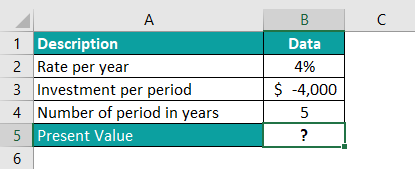Step 2: Next, enter the PV formula in cell B5 to calculate the Present Value of Investment.

So, the entered formula is =PV(B2/12,B4*12,B3,0,0).

Clearly, we can see the result \$2,17,196.28 in the table.

The interest rate is divided by 12, and the period is multiplied by 12 because the investment is made every month, and we need to calculate the Present Value according to the year.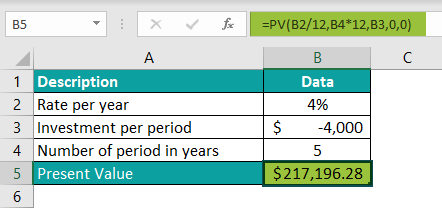Thus, we can obtain results using PV in excel.

How to do PV in Excel?

We can calculate results using PV in Excel. The steps used to calculate PV in excel are:

● First, select the cell.
● Next, type the formula =PV(….
● Select the rate, nper, pmt, fv, and type.
● Press Enter.

Where is the PV function in Excel?

The steps required to use PV Excel functions are:

● First, select the cell which has the number.
● Next, select the Formulas tab.
● Then, click on the Financial option from the Function Library group.● Next, select the PV option from the drop-down list.● The Function Arguments window pops up.
● Next, enter the value in the Rate, Nper, Pmt, Fv, and Type as the number of arguments.
● Click OK.Likewise, we can calculate PV in excel.